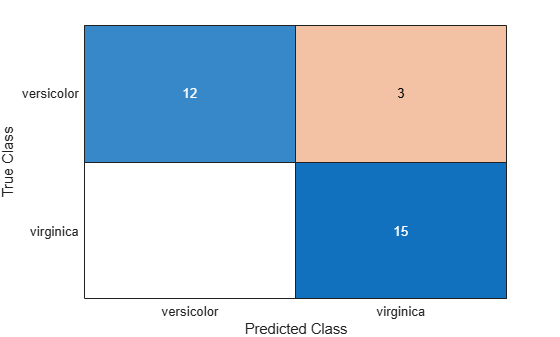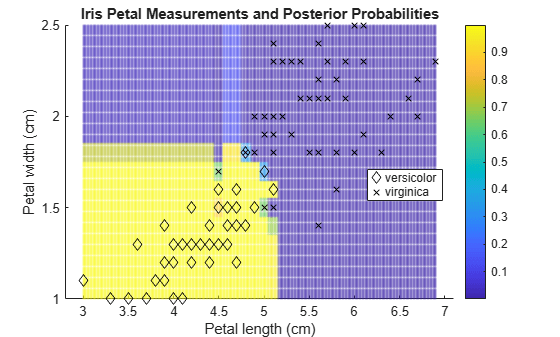predict

Classify observations using generalized additive model (GAM)

Description

example

label = predict(Mdl,X) returns a vector of Predicted Class Labels for the predictor data in the table or matrix X, based on the generalized additive model Mdl for binary classification. The trained model can be either full or compact.

For each observation in X, the predicted class label corresponds to the minimum Expected Misclassification Cost.

example

label = predict(Mdl,X,'IncludeInteractions',includeInteractions) specifies whether to include interaction terms in computations.

example

[label,score] = predict(___) also returns classification scores using any of the input argument combinations in the previous syntaxes.

Examples

collapse all

Train a generalized additive model using training samples, and then label the test samples.

Load the fisheriris data set. Create X as a numeric matrix that contains sepal and petal measurements for versicolor and virginica irises. Create Y as a cell array of character vectors that contains the corresponding iris species.

inds = strcmp(species,'versicolor') | strcmp(species,'virginica');
X = meas(inds,:);
Y = species(inds,:);

Randomly partition observations into a training set and a test set with stratification, using the class information in Y. Specify a 30% holdout sample for testing.

rng('default') % For reproducibility
cv = cvpartition(Y,'HoldOut',0.30);

Extract the training and test indices.

trainInds = training(cv);
testInds = test(cv);

Specify the training and test data sets.

XTrain = X(trainInds,:);
YTrain = Y(trainInds);
XTest = X(testInds,:);
YTest = Y(testInds);

Train a generalized additive model using the predictors XTrain and class labels YTrain. A recommended practice is to specify the class names.

Mdl = fitcgam(XTrain,YTrain,'ClassNames',{'versicolor','virginica'})
Mdl =
ClassificationGAM
ResponseName: 'Y'
CategoricalPredictors: []
ClassNames: {'versicolor'  'virginica'}
ScoreTransform: 'logit'
Intercept: -1.1090
NumObservations: 70

Properties, Methods

Mdl is a ClassificationGAM model object.

Predict the test sample labels.

label = predict(Mdl,XTest);

Create a table containing the true labels and predicted labels. Display the table for a random set of 10 observations.

t = table(YTest,label,'VariableNames',{'True Label','Predicted Label'});
idx = randsample(sum(testInds),10);
t(idx,:)
ans=10×2 table
True Label      Predicted Label
______________    _______________

{'virginica' }    {'virginica' }
{'virginica' }    {'virginica' }
{'versicolor'}    {'virginica' }
{'virginica' }    {'virginica' }
{'virginica' }    {'virginica' }
{'versicolor'}    {'versicolor'}
{'versicolor'}    {'versicolor'}
{'versicolor'}    {'versicolor'}
{'versicolor'}    {'versicolor'}
{'virginica' }    {'virginica' }

Create a confusion chart from the true labels YTest and the predicted labels label.

cm = confusionchart(YTest,label);Estimate the logit of posterior probabilities for new observations using a classification GAM that contains both linear and interaction terms for predictors. Classify new observations using a memory-efficient model object. Specify whether to include interaction terms when classifying new observations.

Load the ionosphere data set. This data set has 34 predictors and 351 binary responses for radar returns, either bad ('b') or good ('g').

Partition the data set into two sets: one containing training data, and the other containing new, unobserved test data. Reserve 10 observations for the new test data set.

rng('default') % For reproducibility
n = size(X,1);
newInds = randsample(n,10);
inds = ~ismember(1:n,newInds);
XNew = X(newInds,:);
YNew = Y(newInds);

Train a GAM using the predictors X and class labels Y. A recommended practice is to specify the class names. Specify to include the 10 most important interaction terms.

Mdl = fitcgam(X(inds,:),Y(inds),'ClassNames',{'b','g'},'Interactions',10);

Mdl is a ClassificationGAM model object.

Conserve memory by reducing the size of the trained model.

CMdl = compact(Mdl);
whos('Mdl','CMdl')
Name      Size              Bytes  Class                                                 Attributes

CMdl      1x1             1081091  classreg.learning.classif.CompactClassificationGAM
Mdl       1x1             1282650  ClassificationGAM

CMdl is a CompactClassificationGAM model object.

Predict the labels using both linear and interaction terms, and then using only linear terms. To exclude interaction terms, specify 'IncludeInteractions',false. Estimate the logit of posterior probabilities by specifying the ScoreTransform property as 'none'.

CMdl.ScoreTransform = 'none';
[labels,scores] = predict(CMdl,XNew);
[labels_nointeraction,scores_nointeraction] = predict(CMdl,XNew,'IncludeInteractions',false);
t = table(YNew,labels,scores,labels_nointeraction,scores_nointeraction, ...
'VariableNames',{'True Labels','Predicted Labels','Scores' ...
'Predicted Labels Without Interactions','Scores Without Interactions'})
t=10×5 table
True Labels    Predicted Labels          Scores          Predicted Labels Without Interactions    Scores Without Interactions
___________    ________________    __________________    _____________________________________    ___________________________

{'g'}            {'g'}           -40.23      40.23                    {'g'}                        -37.484     37.484
{'g'}            {'g'}          -41.215     41.215                    {'g'}                        -38.737     38.737
{'g'}            {'g'}          -44.413     44.413                    {'g'}                        -42.186     42.186
{'g'}            {'b'}           3.0658    -3.0658                    {'b'}                         1.4338    -1.4338
{'g'}            {'g'}          -84.637     84.637                    {'g'}                        -81.269     81.269
{'g'}            {'g'}           -27.44      27.44                    {'g'}                        -24.831     24.831
{'g'}            {'g'}          -62.989     62.989                    {'g'}                          -60.4       60.4
{'g'}            {'g'}          -77.109     77.109                    {'g'}                        -75.937     75.937
{'g'}            {'g'}          -48.519     48.519                    {'g'}                        -47.067     47.067
{'g'}            {'g'}          -56.256     56.256                    {'g'}                        -53.373     53.373

The predicted labels for the test data Xnew do not vary depending on the inclusion of interaction terms, but the estimated score values are different.

Train a generalized additive model, and then plot the posterior probability regions using the probability values of the first class.

Load the fisheriris data set. Create X as a numeric matrix that contains two petal measurements for versicolor and virginica irises. Create Y as a cell array of character vectors that contains the corresponding iris species.

inds = strcmp(species,'versicolor') | strcmp(species,'virginica');
X = meas(inds,3:4);
Y = species(inds,:);

Train a generalized additive model using the predictors X and class labels Y. A recommended practice is to specify the class names.

Mdl = fitcgam(X,Y,'ClassNames',{'versicolor','virginica'});

Mdl is a ClassificationGAM model object.

Define a grid of values in the observed predictor space.

xMax = max(X);
xMin = min(X);
x1 = linspace(xMin(1),xMax(1),250);
x2 = linspace(xMin(2),xMax(2),250);
[x1Grid,x2Grid] = meshgrid(x1,x2);

Predict the posterior probabilities for each instance in the grid.

[~,PosteriorRegion] = predict(Mdl,[x1Grid(:),x2Grid(:)]);

Plot the posterior probability regions using the probability values of the first class 'versicolor'.

h = scatter(x1Grid(:),x2Grid(:),1,PosteriorRegion(:,1));
h.MarkerEdgeAlpha = 0.3;

Plot the training data.

hold on
gh = gscatter(X(:,1),X(:,2),Y,'k','dx');
title('Iris Petal Measurements and Posterior Probabilities')
xlabel('Petal length (cm)')
ylabel('Petal width (cm)')
legend(gh,'Location','Best')
colorbar
hold offInput Arguments

collapse all

Generalized additive model, specified as a ClassificationGAM or CompactClassificationGAM model object.

Predictor data, specified as a numeric matrix or table.

Each row of X corresponds to one observation, and each column corresponds to one variable.

• For a numeric matrix:

• The variables that make up the columns of X must have the same order as the predictor variables that trained Mdl.

• If you trained Mdl using a table, then X can be a numeric matrix if the table contains all numeric predictor variables.

• For a table:

• If you trained Mdl using a table (for example, Tbl), then all predictor variables in X must have the same variable names and data types as those in Tbl. However, the column order of X does not need to correspond to the column order of Tbl.

• If you trained Mdl using a numeric matrix, then the predictor names in Mdl.PredictorNames and the corresponding predictor variable names in X must be the same. To specify predictor names during training, use the 'PredictorNames' name-value argument. All predictor variables in X must be numeric vectors.

• X can contain additional variables (response variables, observation weights, and so on), but predict ignores them.

• predict does not support multicolumn variables or cell arrays other than cell arrays of character vectors.

Data Types: table | double | single

Flag to include interaction terms of the model, specified as true or false.

The default includeInteractions value is true if Mdl contains interaction terms. The value must be false if the model does not contain interaction terms.

Data Types: logical

Output Arguments

collapse all

Predicted Class Labels, returned as a categorical or character array, logical or numeric vector, or cell array of character vectors.

If Mdl.ScoreTransform is 'logit'(default), then each entry of label corresponds to the class with the minimal Expected Misclassification Cost for the corresponding row of X. Otherwise, each entry corresponds to the class with the maximal score.

label has the same data type as the observed class labels that trained Mdl, and its length is equal to the number of rows in X. (The software treats string arrays as cell arrays of character vectors.)

Predicted posterior probabilities or class scores, returned as a two-column numeric matrix with the same number of rows as X. The first and second columns of score contain the first class (or negative class, Mdl.ClassNames(1)) and second class (or positive class, Mdl.ClassNames(2)) score values for the corresponding observations, respectively.

If Mdl.ScoreTransform is 'logit'(default), then the score values are posterior probabilities. If Mdl.ScoreTransform is 'none', then the score values are the logit of posterior probabilities. The software provides several built-in score transformation functions. For more details, see the ScoreTransform property of Mdl.

You can change the score transformation by specifying the 'ScoreTransform' argument of fitcgam during training, or by changing the ScoreTransform property after training.

collapse all

Predicted Class Labels

predict classifies by minimizing the expected misclassification cost:

$\stackrel{^}{y}=\underset{y=1,...,K}{\mathrm{arg}\mathrm{min}}\sum _{j=1}^{K}\stackrel{^}{P}\left(j|x\right)C\left(y|j\right),$

where:

• $\stackrel{^}{y}$ is the predicted classification.

• K is the number of classes.

• $\stackrel{^}{P}\left(j|x\right)$ is the posterior probability of class j for observation x.

• $C\left(y|j\right)$ is the cost of classifying an observation as y when its true class is j.

Expected Misclassification Cost

The expected misclassification cost per observation is an averaged cost of classifying the observation into each class.

Suppose you have Nobs observations that you want to classify with a trained classifier, and you have K classes. You place the observations into a matrix X with one observation per row.

The expected cost matrix CE has size Nobs-by-K. Each row of CE contains the expected (average) cost of classifying the observation into each of the K classes. CE(n,k) is

$\sum _{i=1}^{K}\stackrel{^}{P}\left(i|X\left(n\right)\right)C\left(k|i\right),$

where:

• K is the number of classes.

• $\stackrel{^}{P}\left(i|X\left(n\right)\right)$ is the posterior probability of class i for observation X(n).

• $C\left(k|i\right)$ is the true misclassification cost of classifying an observation as k when its true class is i.

True Misclassification Cost

The true misclassification cost is the cost of classifying an observation into an incorrect class.

You can set the true misclassification cost per class by using the 'Cost' name-value argument when you create the classifier. Cost(i,j) is the cost of classifying an observation into class j when its true class is i. By default, Cost(i,j)=1 if i~=j, and Cost(i,j)=0 if i=j. In other words, the cost is 0 for correct classification and 1 for incorrect classification.